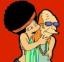# Strukovni Humor

## Recommended PostsHahaha, znam jednog koji je spoj OCD i teoretskog programera :D

Fali "mindless code-machine programmer" ili tako nesto, znao sam par takvih, sednu i pocnu da pisu vrlo komplikovan program bez da su 5 sekundi razmislili ista o strukturi: "So we need to do a peer-to-peer thing, uh? So we'll need a hash map for that..." i samo pocne. I tako. I onda posle 15 minuta imas 500 linija koda, potpuno beskorisnih

Edited by hazardPfft.

sudo apt-get schwarzwald-cake-full:D

Zapravo CSS ali da ne sitnicarimo.pff...

```#include <stdio.h>
int main() {
float b[] = {1.1431391224375825e+27, 6.6578486920496456e+28, 7.7392930965627798e-19, 3.2512161851627752e-9};
printf("%s",(char*)b);
return 0;
}
```
edit: ili pak

```#include <stdio.h>
#include <stdlib.h>
#define u unsigned char
#define v while(*x)
#define z(x) putchar(*x);
#define y(x) ++*x;
#define p(x) ++x;
#define e(x) --*x;
#define d(x) --x;
#define w(x) x x
main(){u *x=calloc(12,1);u *l=x;w(w(w(y(x))))w(y(x))v{p(x)w(w(y(x)))w(y(x))y(x)p
(x)w(w(w(y(x))))w(y(x))p(x)w(y(x))y(x)p(x)w(w(w(y(x))))y(x)w(w(d(x)))e(x)}p(x)w(
y(x))z(x)p(x)y(x)z(x)w(w(y(x)))w(y(x))y(x)w(z(x))w(y(x))y(x)z(x)p(x)w(y(x))z(x)p
(x)w(e(x))e(x)z(x)w(d(x))z(x)w(y(x))y(x)z(x)w(w(e(x)))w(e(x))z(x)w(w(w(e(x))))z(
x)p(x)y(x)free(l);}
```
Edited by Clem FandangoA typical conversation

Person: What do you do?

Me: I am a computer science professor.

Person: I have a problem with my PC, can you fix it?

Me: No, I don’t think I can do that.

Person: You will not fix my PC?

Me: I cannot fix my PC, let alone yours.

Person: Then what exactly do you do?

Me: I study algorithms.

Person: Oh, I know that.

Me: Really?

Person: Yes! I learnt logarithms in high school.

Edited by slow

## Join the conversation

You can post now and register later. If you have an account, sign in now to post with your account.×   Pasted as rich text.   Paste as plain text instead

Only 75 emoji are allowed.

×   Your previous content has been restored.   Clear editor

×   You cannot paste images directly. Upload or insert images from URL.

×

×